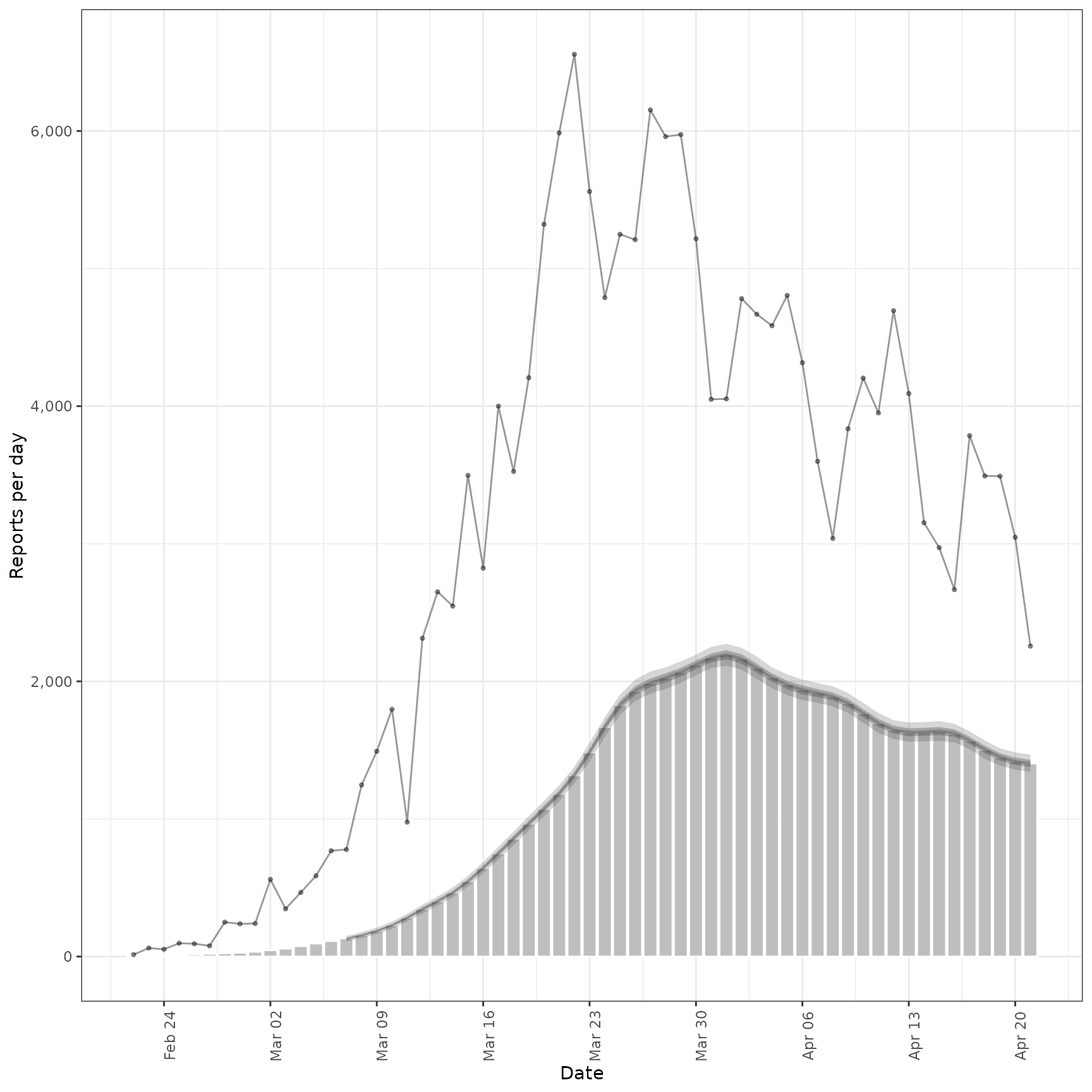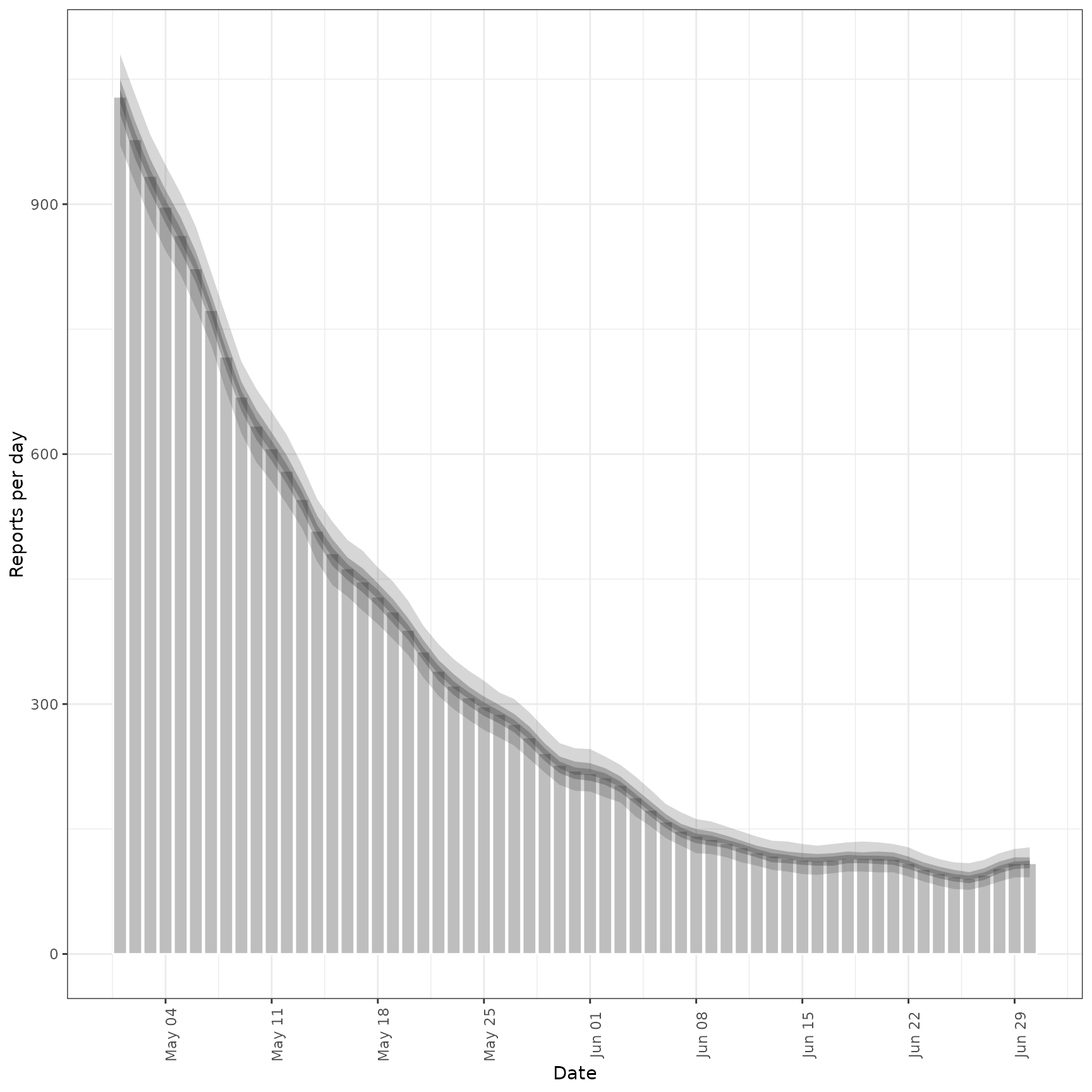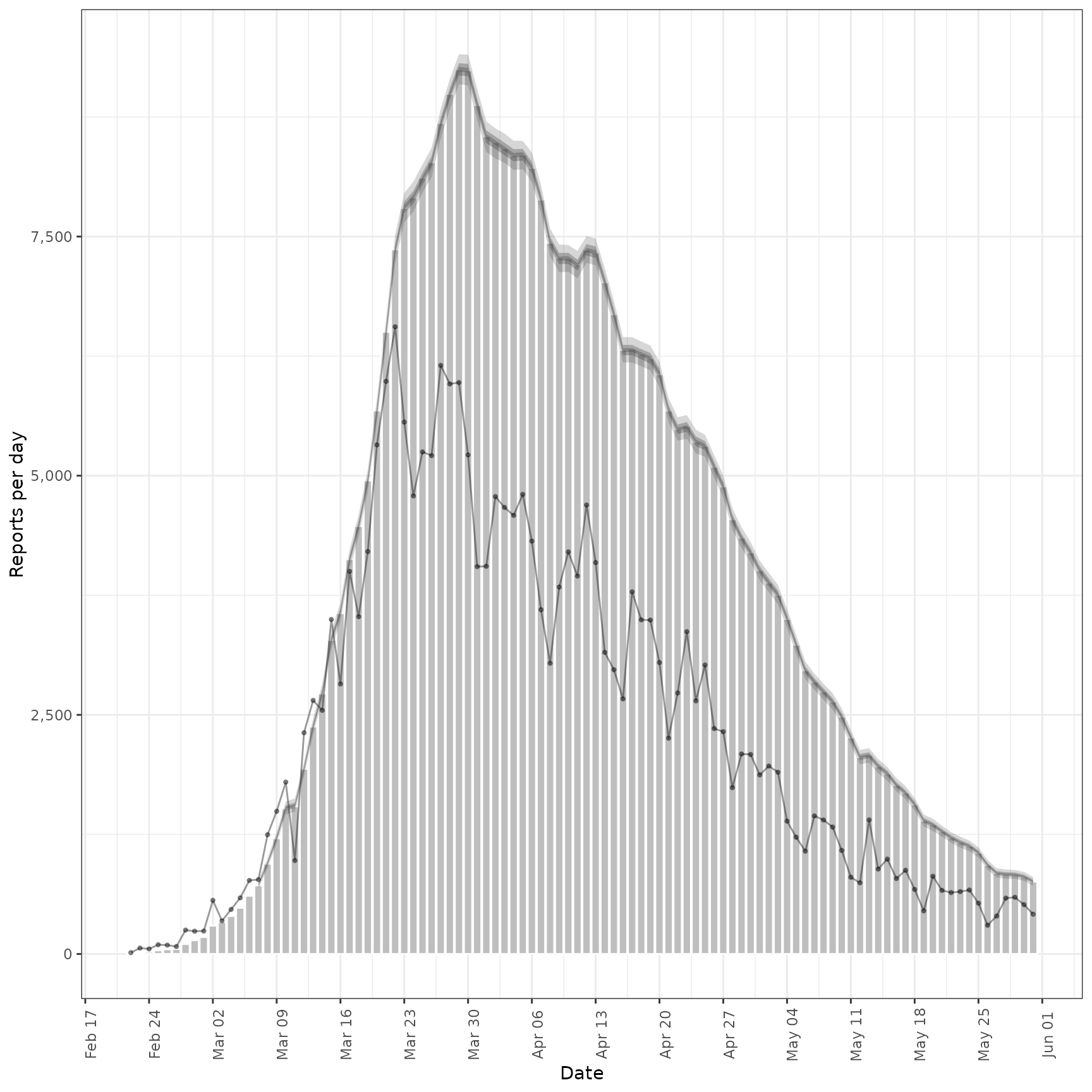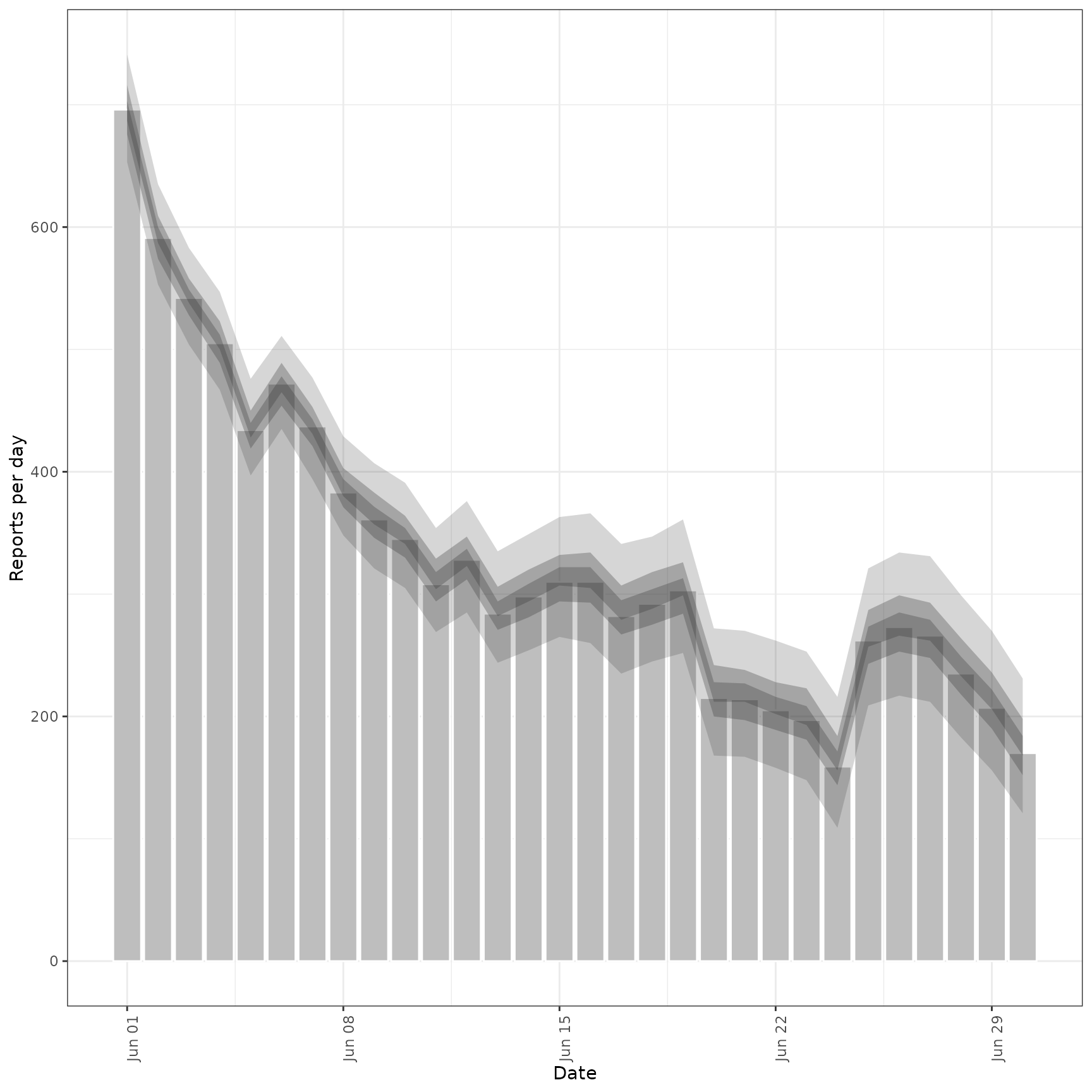Estimates the relationship between a primary and secondary observation, for example hospital admissions and deaths or hospital admissions and bed occupancy. See secondary_opts() for model structure options. See parameter documentation for model defaults and options. See the examples for case studies using synthetic data and here for an example of forecasting Covid-19 deaths from Covid-19 cases. See here for a prototype function that may be used to estimate and forecast a secondary observation from a primary across multiple regions and here for an application forecasting Covid-19 deaths in Germany and Poland.

estimate_secondary(
reports,
secondary = secondary_opts(),
delays = delay_opts(list(mean = 2.5, mean_sd = 0.5, sd = 0.47, sd_sd = 0.25, max =
30)),
truncation = trunc_opts(),
obs = obs_opts(),
burn_in = 14,
CrIs = c(0.2, 0.5, 0.9),
model = NULL,
verbose = interactive(),
...
)

## Arguments

reports

A data frame containing the date of report and both primary and secondary reports.

secondary

A call to secondary_opts() or a list containing the following binary variables: cumulative, historic, primary_hist_additive, current, primary_current_additive. These parameters control the structure of the secondary model, see secondary_opts() for details.

delays

A call to delay_opts() defining delay distributions between primary and secondary observations See the documentation of delay_opts() for details. BY default a diffuse prior is assumed with a mean of 14 days and standard deviation of 7 days (with a standard deviation of 0.5 and 0.25 respectively on the log scale).

truncationA list of options as generated by trunc_opts() defining the truncation of observed data. Defaults to trunc_opts(). See estimate_truncation() for an approach to estimating truncation from data.

obs

A list of options as generated by obs_opts() defining the observation model. Defaults to obs_opts().

burn_in

Integer, defaults to 14 days. The number of data points to use for estimation but not to fit to at the beginning of the time series. This must be less than the number of observations.

CrIs

Numeric vector of credible intervals to calculate.

model

A compiled stan model to override the default model. May be useful for package developers or those developing extensions.

verbose

Logical, should model fitting progress be returned. Defaults to interactive().

...

Additional parameters to pass to rstan::sampling.

## Value

A list containing: predictions (a data frame ordered by date with the primary, and secondary observations, and a summary of the model estimated secondary observations), data (a list of data used to fit the model), and fit (the stanfit object).

## Examples

# \donttest{
# set number of cores to use
options(mc.cores = ifelse(interactive(), 4, 1))
#' # load data.table for manipulation
library(data.table)
library(lubridate)
#>
#> Attaching package: ‘lubridate’
#> The following objects are masked from ‘package:data.table’:
#>
#>     hour, isoweek, mday, minute, month, quarter, second, wday, week,
#>     yday, year
#> The following objects are masked from ‘package:base’:
#>
#>     date, intersect, setdiff, union
library(purrr)
#>
#> Attaching package: ‘purrr’
#> The following object is masked from ‘package:data.table’:
#>
#>     transpose
#> The following object is masked from ‘package:EpiNow2’:
#>
#>     update_list

#### Incidence data example ####

# make some example secondary incidence data
cases <- example_confirmed
cases <- as.data.table(cases)

# apply a convolution of a log normal to a vector of observations
weight_cmf <- function(x, ...) {
set.seed(x)
meanlog <- rnorm(1, 1.6, 0.2)
sdlog <- rnorm(1, 0.8, 0.1)
cmf <- cumsum(dlnorm(1:length(x), meanlog, sdlog)) -
cumsum(dlnorm(0:(length(x) - 1), meanlog, sdlog))
cmf <- cmf / plnorm(length(x), meanlog, sdlog)
conv <- sum(x * rev(cmf), na.rm = TRUE)
conv <- round(conv, 0)
return(conv)
}
# roll over observed cases to produce a convolution
cases <- cases[, .(date, primary = confirm, secondary = confirm)]
cases <- cases[, secondary := frollapply(secondary, 15, weight_cmf, align = "right")]
cases <- cases[!is.na(secondary)]
# add a day of the week effect and scale secondary observations at 40\% of primary
cases <- cases[lubridate::wday(date) == 1, secondary := round(0.5 * secondary, 0)]
cases <- cases[, secondary := round(secondary * rnorm(.N, 0.4, 0.025), 0)]
cases <- cases[secondary < 0, secondary := 0]
cases <- cases[, secondary := map_dbl(secondary, ~ rpois(1, .))]

# fit model to example data assuming only a given fraction of primary observations
# become secondary observations
inc <- estimate_secondary(cases[1:60],
obs = obs_opts(scale = list(mean = 0.2, sd = 0.2))
)
plot(inc, primary = TRUE)# forecast future secondary cases from primary
inc_preds <- forecast_secondary(inc, cases[61:.N][, value := primary])
plot(inc_preds, new_obs = cases, from = "2020-05-01")#### Prevalence data example ####

# make some example prevalence data
cases <- example_confirmed
cases <- as.data.table(cases)
cases <- cases[, .(date,
primary = confirm,
scaled_primary = confirm * rnorm(.N, 0.4, 0.05)
)]
cases$secondary <- 0 cases$secondary <- as.integer(cases$scaled_primary) for (i in 2:nrow(cases)) { meanlog <- rnorm(1, 1.6, 0.1) sdlog <- rnorm(1, 0.8, 0.05) cmf <- cumsum(dlnorm(1:min(i - 1, 20), meanlog, sdlog)) - cumsum(dlnorm(0:min(19, i - 2), meanlog, sdlog)) cmf <- cmf / plnorm(min(i - 1, 20), meanlog, sdlog) reducing_cases <- sum(cases$scaled_primary[(i - 1):max(1, i - 20)] * cmf)
reducing_cases <- ifelse(cases$secondary[i - 1] < reducing_cases, cases$secondary[i - 1], reducing_cases
)
cases$secondary[i] <- as.integer( cases$secondary[i - 1] + cases$scaled_primary[i] - reducing_cases ) cases$secondary[i] <- ifelse(cases$secondary[i] < 0, 0, cases$secondary[i]
)
}
cases <- cases[, secondary := map_dbl(secondary, ~ rpois(1, .))]

# fit model to example prevalence data
prev <- estimate_secondary(cases[1:100],
secondary = secondary_opts(type = "prevalence"),
obs = obs_opts(
week_effect = FALSE,
scale = list(mean = 0.3, sd = 0.1)
)
)
plot(prev, primary = TRUE)# forecast future secondary cases from primary
prev_preds <- forecast_secondary(prev, cases[101:.N][, value := primary])
plot(prev_preds, new_obs = cases, from = "2020-06-01")# }